# How to Flip or Reverse Data in Excel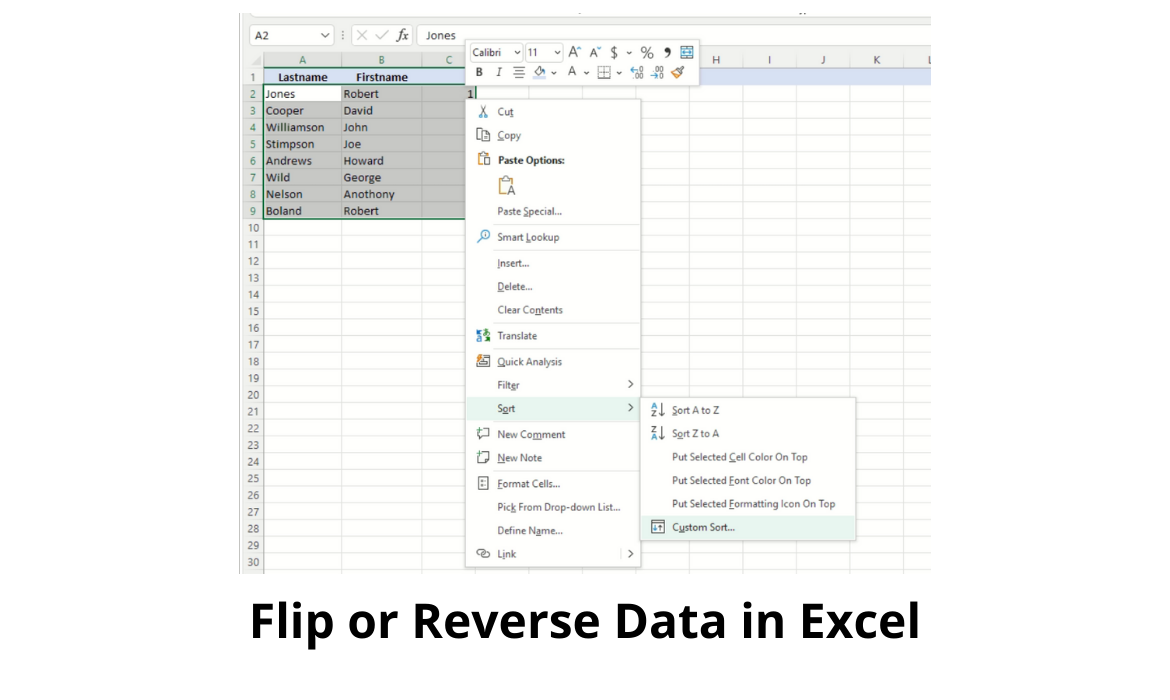Today we’ll be learning How to Flip or Reverse Data in Excel, which refers to the process of reversing the current order of a list or an array of data.

This would be a piece of cake in Excel if the data is ordered, but what if it isn’t?

While Excel is excellent at reverse sorting, if the data is not already sorted, there is no menu option or function to flip the data.

Along the path to solving this problem, we will also take a look at how to flip data in a row.

## Flip (or Reverse) Data in Excel

But first, let’s take a look at how to flip data in a column or columns.

We will be using the following simple list of names.

Our aim is to flip on the Lastname column.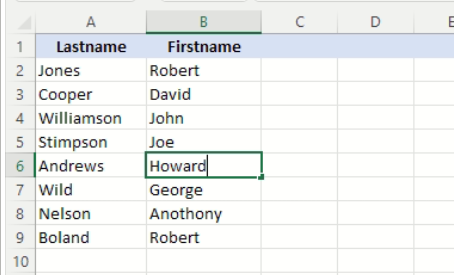As we can see, the names are not sorted, but the first method we will employ will rely on sorting, just not on the Lastname column.

Instead, we are going to turn column C into an auto-incrementing counter.

In this case, column C is referred to as a helper column.

It serves no purpose other than to take on the role of a sorted column, and in fact, once we’ve achieved our goal, we can delete it.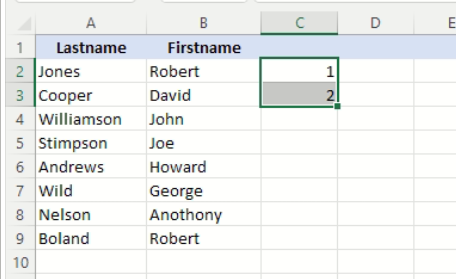We start by entering the number 1 into row 2 of column C, and the number 2 into row 3 of the same column.

This sets the pattern, and since Excel is smart enough to know what we want, we can then use the fill handle at the bottom right hand corner of the bounding box around cells C2 and C3, to drag it down to cell C9, thus populating the remaining rows in column C.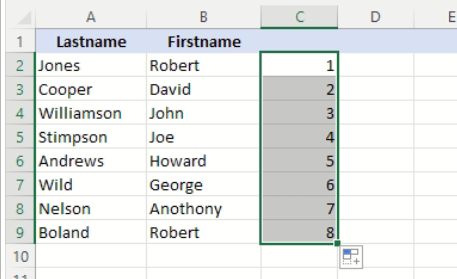The result is that we have now filled column C with auto-incrementing numbers.

Dragging the fill handle may not seem much of a time saver with so few entries, but imagine having to enter the numbers manually in a sheet comprising hundreds of rows.

Column C is now a sorted column, which means we can easily reverse the order of our sheet based on this column, using the built-in sort functionality.We start by selecting all the cells that will be included in the sort, which in our case is the range of cells A2 to C9.

Next, we right mouse click inside the selected cell range to bring up the context menu, and select Sort > Custom Sort…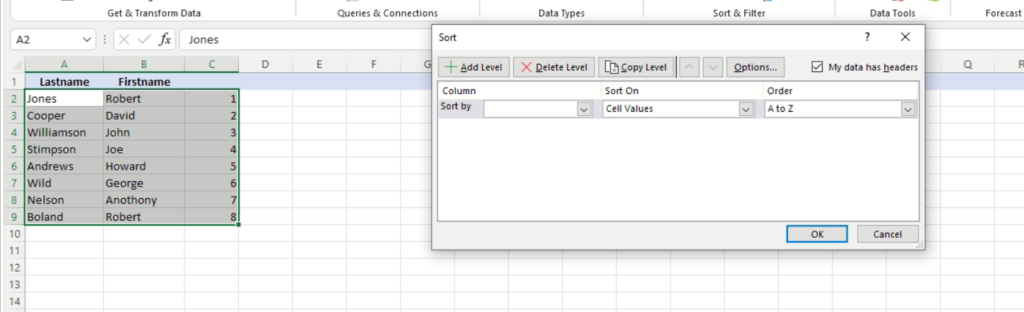This will open the Sort dialog box. We now need to specify the criteria by which to sort.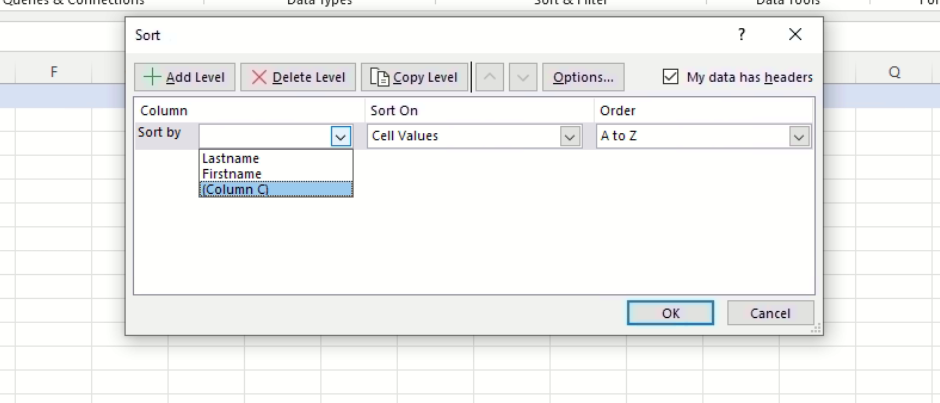First, we specify on which column we will sort, in our case Column C.

We can leave the Sort On field as is, Cell Values.Since we want to flip the order of our data, we also need to change the Order field from Smallest to Largest to Largest to Smallest so that the order in column C results in 8 at the top, down to 1 at the bottom.Once these are set, click on the OK button to apply the sort.

The result, as can be seen in the screenshot above, is that the order of the names is now in reverse order to that originally.

The next method of reversing the order, involves the use of a couple of Excel functions.

There is however, one consideration to take note of.

Whereas the previous method flipped the original columns directly, this method creates a new column or columns (depending on the number of columns included in our sort), that contain the flipped data, leaving the original columns intact.

The functions that we are going to be using are INDEX and ROWS. Their definitions are given below.

## INDEX

The INDEX function returns a value or the reference to a value from within a table or range.

### Syntax

INDEX(array, row_num, [column_num])

The array form of the INDEX function has the following arguments:

• array    Required. A range of cells or an array constant.
• If array contains only one row or column, the corresponding row_num or column_num argument is optional.
• If array has more than one row and more than one column, and only row_num or column_num is used, INDEX returns an array of the entire row or column in array.
• row_num    Required, unless column_num is present. Selects the row in array from which to return a value. If row_num is omitted, column_num is required.
• column_num    Optional. Selects the column in array from which to return a value. If column_num is omitted, row_num is required.

### Sample Usage

INDEX(A1:C20, 5, 1)

## ROWS

Returns the number of rows in a specified array or range.

### Syntax

ROWS(range)

• range – The range whose row count will be returned

### Remarks

• If both the row_num and column_num arguments are used, INDEX returns the value in the cell at the intersection of row_num and column_num.
• row_num and column_num must point to a cell within array; otherwise, INDEX returns a #REF! error.
• If you set row_num or column_num to 0 (zero), INDEX returns the array of values for the entire column or row, respectively. To use values returned as an array, enter the INDEX function as an array formula.

### Sample Usage

ROWS(A9:A62)

ROWS({1;2;3;4;5})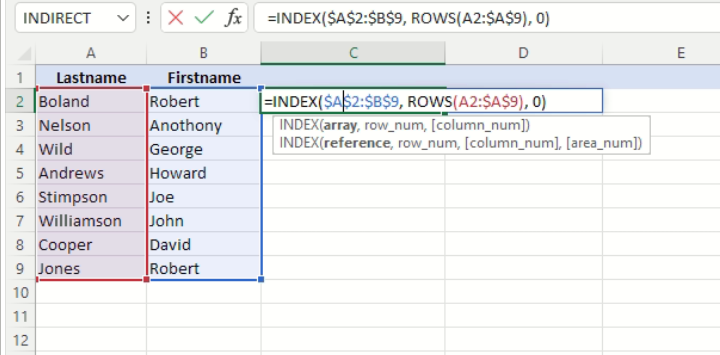Our formula is =INDEX(\$A\$2:\$B\$9, ROWS(A2:\$A\$9), 0) which we enter into the empty cell C2, as shown in the snapshot above.

The ROWS function call returns the number of rows in the range passed, which is initially set to A2:\$A\$9.

The first part of the range, A2, is a relative cell reference, while the second part, \$A\$9, is an absolute cell reference.

The reason for this is that we will use the fill handle to propagate our formula into all the cells from C2 to C9 in our sheet, and we want the first part of the range to be automatically adjusted to match the row number.

For cell C2, ROWS will return the number of cells from A2 to A9, which is 7.

For cell C3, Excel will have adjusted the formula so that the cell reference will now be A3:\$A\$9.

Notice that the relative reference has been updated to A3, while the absolute reference has remained fixed at A9.

So, for cell C3, the ROWS function will return the number of rows from A3 to A9, which is 6.

For cell C4, ROWS will return the number of rows for the range A4:\$A\$9, which is 5, and so on down the column.

Hence, the ROWS function is returning a decreasing count, starting with 7 for cell C2, and going down to 1 for cell C9.

The INDEX function accepts a range as its first parameter, and returns the cell in the range referenced by the row and column indexes supplied as the second and third parameters respectively.

Our range is fixed at \$A\$2:\$B\$9.

The row index is supplied by the ROWS function call, which for cell C2 will be 7, while we have hard set the column index to 0 and this deserves a short explanation.

Normally, the index parameter has a value of 1 or greater, and references which column in a range is to be referenced, the first column being 1.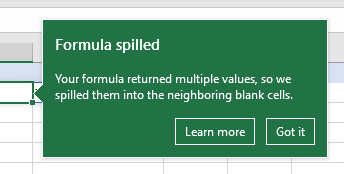Specifying a column index of 0 causes the INDEX function to return what Excel calls the results of a spilled formula, which returns the entire row in the range.

If we change the column index parameter to 1 in the formula, we would only get the Lastname column’s cell being returned, or 2 for only the Firstname.

As we have it, we get the entire row in the range.

You can read more on spilled array behavior in the Microsoft support article Dynamic array formulas and spilled array behavior.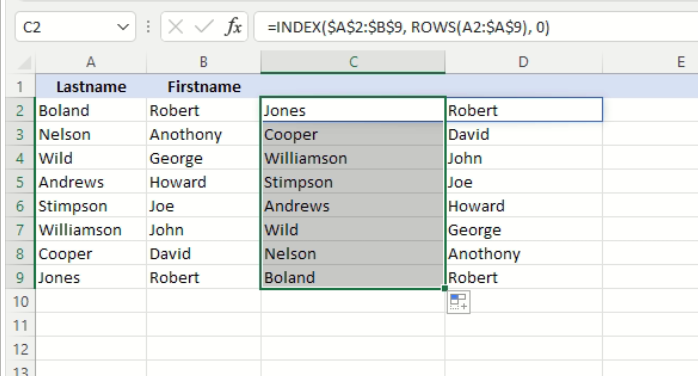Having entered the formula, we can now apply it to the remaining cells in column C by dragging the fill handle down to cell C9.

The result is as shown above, where columns C and D are the flip of the original columns A and B.

## Flip Row Data in Excel

Unfortunately, Excel sorts data only by columns, not by rows, so we can’t call upon the Sort > Custom Sort… menu option.

We can however, use our INDEX formula with one minor change.

Instead of using the ROWS function, we use the equivalent, COLUMNS function.Let’s say we want to horizontally reverse (or flip) the order of the days and names in the rows shown in the sheet above.

To do this, we can adapt our formula used to flip our column data, to do the same for rows. Instead of ROWS, we used COLUMNS to return the number of columns in our range.

The definition of the COLUMNS function is given below.

## COLUMNS

Returns the number of columns in an array or reference.

### Sample Usage

COLUMNS(A9:W62)

COLUMNS({1,2,3,4,5})

### Syntax

COLUMNS(array)

The COLUMNS function syntax has the following argument:

• Array    Required. An array or array formula, or a reference to a range of cells for which you want the number of columns.Our formula now becomes =INDEX(\$A\$1:\$G\$2,0, COLUMNS(A1:\$G\$2)), where the COLUMNS function counts how many rows from A1 (the leftmost row) to \$G\$2 (the rightmost row).

Note also, that the row index, the second parameter in the INDEX function call, is explicitly set to 0, so that we flip both row 1 and row 2.Using the fill handle, we can propagate the formula to the remaining cells in the row, up to cell G4.

Excel will once again adjust any relative cell references in the formula.

The result is we have in rows 4 and 5, the reverse of rows 1 and 2.

## Conclusion

As we’ve seen, Excel can only directly sort columns, and even then they must already be sorted.

However, with a bit of ingenuity using a helper column, we can sort data that has no sort order.

This method will sort the original data directly.

We can also sort with a formula with function calls, specifically, using the INDEX and ROWS functions.

This way, we leave the original column data intact and create new columns that contain the flipped data.

Excel cannot sort row data, even with a helper row.

Excel’s sort functionality only works on columns.

However, a formula can be used, but this time using the INDEX and COLUMNS functions.

As with columns, the formula method will create additional rows with the flipped data and the original rows left intact.

As always, there are enough tools and functionality built in to Excel to achieve even the most defiant of tasks. We hope this tutorial on How to Flip or Reverse Data in Excel was helpful to finishing your task!

Costas has a wide range of experience in Information Technology covering computer hardware, programming, telecommunications, networking, web services, and general IT support. He's worked in various roles such as PHP programmer and web developer, technical and desktop support, hardware repair, system administration. Costas has excellent background in Microsoft Windows and Office Suite (Excel, Word, Powerpoint, etc), as well as a thorough understanding of Networking and Hardware maintenance.